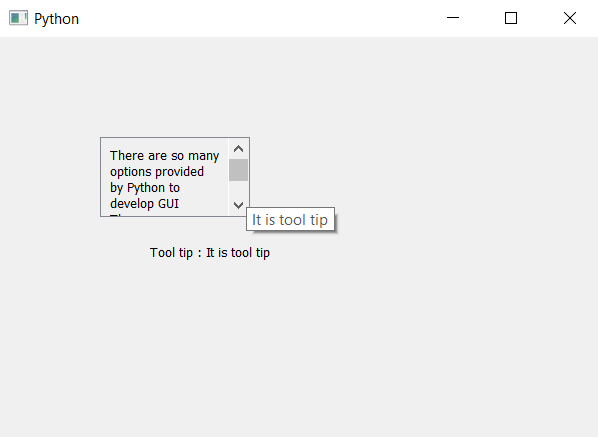# PyQt5 Scrollable Label – Retrieving tooltip text

• Last Updated : 03 May, 2020

In this article we will see how we can get tooltip text of the scroll label, when know we can make scrollable label with the help of inheriting a scroll class and making label in it. Scroll Label is basically two widgets combined together scroll area act as a base widget and label act as a component of this class.

Steps for implementations –

1. Create a new class which inherits QScrollArea
2. Inside the class create vertical layout
3. Create a label and make it multi-line and add it to the layout
4. Create object of this class inside the main window class and setting text to it
5. Add tool tip to the object with the help of `setToolTip` method
6. Retrieve the tool tip text with the help of `toolTip` method
7. Create another label to show the tool tip text

Below is the implementation

 `# importing libraries``from` `PyQt5.QtWidgets ``import` `*` `from` `PyQt5 ``import` `QtCore, QtGui``from` `PyQt5.QtGui ``import` `*` `from` `PyQt5.QtCore ``import` `*` `import` `sys`` ` ` ` `# class for scrollable label``class` `ScrollLabel(QScrollArea):`` ` `    ``# constructor``    ``def` `__init__(``self``, ``*``args, ``*``*``kwargs):``        ``QScrollArea.__init__(``self``, ``*``args, ``*``*``kwargs)`` ` `        ``# making widget resizable``        ``self``.setWidgetResizable(``True``)`` ` `        ``# making qwidget object``        ``content ``=` `QWidget(``self``)``        ``self``.setWidget(content)`` ` `        ``# vertical box layout``        ``lay ``=` `QVBoxLayout(content)`` ` `        ``# creating label``        ``self``.label ``=` `QLabel(content)`` ` `        ``# making label multi-line``        ``self``.label.setWordWrap(``True``)`` ` `        ``# adding label to the layout``        ``lay.addWidget(``self``.label)`` ` `    ``# the setText method``    ``def` `setText(``self``, text):``        ``# setting text to the label``        ``self``.label.setText(text)`` ` `    ``# getting text method``    ``def` `text(``self``):``        ``# getting text of the label``        ``get_text ``=` `self``.label.text()`` ` `        ``# return the text``        ``return` `get_text`` ` ` ` `class` `Window(QMainWindow):`` ` `    ``def` `__init__(``self``):``        ``super``().__init__()`` ` `        ``# setting title``        ``self``.setWindowTitle(``"Python "``)`` ` `        ``# setting geometry``        ``self``.setGeometry(``100``, ``100``, ``600``, ``400``)`` ` `        ``# calling method``        ``self``.UiComponents()`` ` `        ``# showing all the widgets``        ``self``.show()`` ` `    ``# method for widgets``    ``def` `UiComponents(``self``):``        ``# text to show in label``        ``text ``=` `"There are so many options provided by Python to develop GUI "` `\``               ``" There are so many options provided by Python to develop GUI"` `\``               ``" There are so many options provided by Python to develop GUI"`` ` `        ``# creating scroll label``        ``label ``=` `ScrollLabel(``self``)`` ` `        ``# setting text to the label``        ``label.setText(text)`` ` `        ``# setting geometry``        ``label.setGeometry(``100``, ``100``, ``150``, ``80``)`` ` `        ``# setting tool tip``        ``label.setToolTip(``"It is tool tip"``)`` ` `        ``# getting the tool tip``        ``tip ``=` `label.toolTip()`` ` `        ``# creating another label to show the tool tip``        ``result ``=` `QLabel(``"Tool tip : "` `+` `tip, ``self``)`` ` `        ``# setting geometry to the label``        ``result.setGeometry(``150``, ``200``, ``200``, ``30``)`` ` ` ` `# create pyqt5 app``App ``=` `QApplication(sys.argv)`` ` `# create the instance of our Window``window ``=` `Window()`` ` `# start the app``sys.exit(App.``exec``())`

Output :My Personal Notes arrow_drop_up